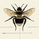# FREE INDICATOR: Relative Momentum Index (RMI)

8136 views
8136
RMI, as requested by glaz

Description:
The Relative Momentum Index was developed by Roger Altman and was introduced in his article in the February, 1993 issue of Technical Analysis of Stocks & Commodities magazine.
While your typical RSI counts up and down days from close to close, the Relative Momentum Index counts up and down days from the close relative to a close x number of days ago. The result is an RSI that is a bit smoother.

Usage:
Use in the same way you would any other RSI . There are overbought and oversold zones, and can also be used for divergence and trend analysis .

Grab the source code here: http://pastebin.com/LRVusQmy
Installation video by @ChrisMoody here : http://vimeopro.com/user14689865/trading...

░░░░░░░░░░░░░░░ Feel free to follow me to keep up with my latest scripts! ░░░░░░░░░░░░░░░
░░░░░░░░░░░░ PLEASE THUMB UP OR STAR IF YOU LIKE THIS INDICATOR! ░░░░░░░░░░░░
I'd like as many people as possible to get it :)
```study(title = "TheLark Relative Momentum Index (RMI)",overlay=false)

//•/•/•/•/•/•/•/•/•/•/•/•/•/•/•/•/•/•/•/•/•/•/•//
//                                             //
//                RMI BY THELARK               //
//                 ~ 2-19-14 ~                 //
//                                             //
//                     •/•                     //
//                                             //
//    https://www.tradingview.com/u/TheLark    //
//                                             //
//•/•/•/•/•/•/•/•/•/•/•/•/•/•/•/•/•/•/•/•/•/•/•//

// Relative Momentum Index (RMI)
// "... The Relative Momentum Index was developed by Roger Altman
// and was introduced in his article in the February, 1993 issue of
// Technical Analysis of Stocks & Commodities magazine. "
// "... While RSI counts up and down days from close to close, the Relative
// Momentum Index counts up and down days from the close relative to a
// close x number of days ago. "

// Requested by glaz @ TradingView

// inputs
len = input(20, title="Length")
mom = input(4, title="Momentum",minval=0)
ob = input(70,title="Overbought")
os = input(30,title="Oversold")
c = close
docol = input(true,title="Change Color?")
dosignal = input(true,title="Show Signal Line?")
sig = input(6,title="Signal Length")
dohist = input(false,title="Show Hist?")
//calc
up = ema(max(c - c[mom],0),len)
dn = ema(max(c[mom] - c,0),len)
rmi = dn == 0 ? 0 : 100 - 100 / (1 + up / dn)
signal = sma(rmi,sig)

//plots
hline(ob)
hline(os)
plot(dohist?(rmi-signal)+50:na,color=#FF006E,histbase=50,style=histogram,linewidth=2)
plot(dosignal?signal:na,color=#D87A68)
col = docol ? rmi > rmi ? #0094FF : #FF006E : #0094FF
plot(rmi, color=col,linewidth=2)```hi Lark, i believe there's an error in how you compute the RMI. on this page https://www.prorealcode.com/prorealtime-indicators/relative-momentum-index-rmi/

i found the formula expressed as:

momup=max(close-close,0)
momdown=max(close-close,0)

up = average(momup)
dn = average(momdown)

rm=up/dn

rmi = 100*rm/(1+rm)

note rmi = 100*rm/(1+rm) and NOT 100 - 100 / (1 + up / dn) as you have it in your code.

this came to my attention when i noticed that your RMI does not always paint the same way on a 15 mins chart as on other platforms.

i'm modifying the code now to see if that results in the same values as in the other platforms.
Replyisomorph
@isomorph, @TheLark nevermind... just realized they are mathematically identical. and yet, the plot output is slightly different, not much, but it's not equal either. weird. i used parans around the higher priority operators just so there is no confusion possible in case the Pine script engine has a bug or treats operator priority differently than in math.

so this: rmi = dn == 0 ? 0 : 100 * (up / dn) / (1 + (up / dn))

does not equal this when plotted: rmi = dn == 0 ? 0 : 100 - 100 / (1 + (up / dn))

WTF!? is going on?
Replyisomorph
@TheLark jesus!!! major brain fart today. sorry, the plots are exactly the same. i need sleep. :( must be the other platforms that are using some other calculation.
Replyisomorph
@isomorph, Funny how diluted a lot of indicators get. It's like a big game of telephone! I always try to read and translate the original paper when I work with known indicators like this :)

Regardless, peer review is always a good thing :)
ReplyHey ThLark, thanks for continuing doing that.

The question relative RSI definition. Several years ago I wrote a piece of code, doing exactly what RSI defines: "... up and down days from close to close...".

If you do it by yourself and plot the indicator (not just using built-in function), you'll see completely different graph.

As I understood, the standard built-in RSI is some sort of approximation to the original definition.
Reply